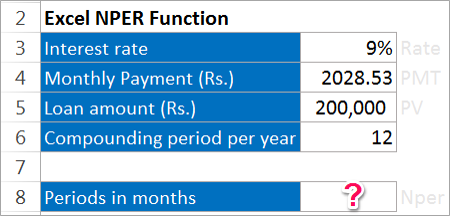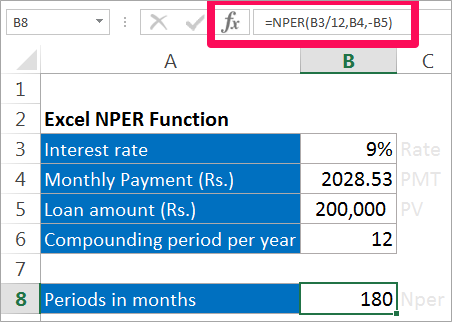Need Help? Chat with us

# NPER function | Nper formulas in Excel in Hindi

## Nper function – Excel Formulas in Hindi

Nper function denotes the time period required to pay off the loans. This function will normally give answer to the question like suppose I have Rs. 200000 today how much time will it take double the money at interest rate of 6%. If we know the rate, Present value and the Annuity Payment we can easily find the number of years.

The formula for calculating Nper function

## Special Offer for First Time Users

If you are the first time user and want to learn Microsoft office skills, then you can avail this special offer discount on your email.NPER(rate, pmt, pv, [fv], [type])

Where,

Nper stands for Number of years or the time period taken.

Rate stands for the interest rate at which is provided.

PMT stands for Payment Annuity.

PV stands for the Present Value.

FV stands for the Future Value.

Type stands for when the payment is made.

The arguments in the box brackets are optional.

### Example of Nper function

Rs 2028.53 is paid monthly for a loan of Rs 200,000. Interest rate is 9% and the payment needs to be done yearly. How much time will it take to pay off the loan.Solution:

Here, Rate = 9%

PMT= Rs 2028.53

PV = Rs 200,000

The other arguments are optional.We will see that time required to pay a loan of Rs.200,000 is 180 Months.

• What is Nper excel
• using nper function
• How to Calculate nper function

Note: PMT includes principal and interest and not taxes, fees or reserve payments.

Hope you like our tutorial on Nper Function for more cool and amazing trick of excel Like our Excel Superstar Facebook Page and subscribe to our Excel Superstar YouTube channel. Excel Superstar is the leading online training company, which provides Online Excel Course in Hindi. Connect with us and become an Excel Superstar.